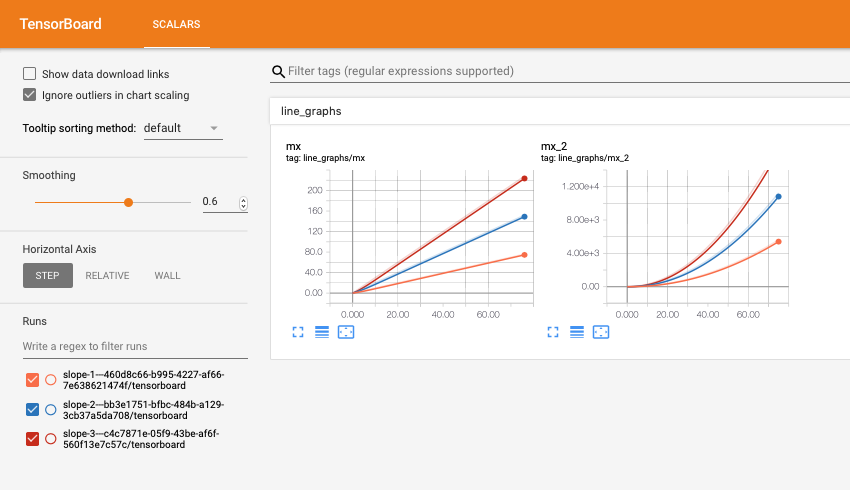# Walkthrough 2: Using cox with tensorboardX¶

Note: As with the first walkthrough, a working example file with all of these commands can be found here

Here, we’ll show how to use `cox` and `tensorboardX` in unison for logging. We’ll use the following simple running example:

```from cox.store import Store

for slope in range(5):
s = Store(OUT_DIR) # Create OUT_DIR/RANDOM_UUID

# GOAL: plot and log the lines "y=slope*x" and "y=slope*x^2"
```

As previously mentioned, `cox.store.Store` objects also automatically creates a `tensorboard` folder that is written to via the tensorboardX library. A created `cox.store.Store` object will actually expose a `writer` property that is a fully functioning SummaryWriter object. That means we can plot the lines we want in TensorBoard as follows:

```for x in range(10):
```

Unfortunately, TensorBoard data is quite hard to read/manipulate through means other than the TensorBoard interface. For convenience, the `Store` object also provides the ability to write to a table and the `tensorboardX` writer at the same time through the `cox.store.Store.log_table_and_tb()` function, meaning that we can replace the above with:

```# Does the same thing as the example above but also stores the results in a
for x in range(10):
s.log_table_and_tb('line_graphs', {'mx': slope*x, 'mx^2': slope*(x**2)})
s['line_graphs'].flush_row()
```

## Viewing multiple tensorboards with cox.tensorboard_view¶

Note: the `python3 -m cox.tensorboard_view` command can be called as `cox-tensorboard` **from the command line

Continuing with our running example, we may now want to visually compare TensorBoards across multiple parameter settings. Fortunately, `cox` provides utilities for comparing TensorBoards across experiments in a readable way. In our example, where we made a `Store` object and a table called `metadata` where we stored hyperparameters. We also showed how to integrate TensorBoard logging via `tensorboardX`. We’ll now use the `cox.tensorboard_view` utility to view the tensorboards from multiple jobs at once (this is useful when comparing parameters for a grid search).

The way to achieve this is through the `cox.tensorboard_view` command, which is called as `python3 -m cox.tensorboard_view` with the following arguments:

`--logdir`: (required)
the directory where all of the stores are located
`--port` (default 6006)
the port on which to run the tensorboard server
`--metadata-table` (default “metadata”)
the name of the table where the hyperparameters are saved (i.e. “metadata” in our running example). This should be a table with a single row, as in our running example.
`--filter-param` (optional)
Can be used more than once, filters out stores from the tensorboard aggregation. For each argument of the form `--filter-param PARAM_NAME PARAM_REGEX`, only the stores where `PARAM_NAME` in the metadata matches `PARAM_REGEX` will be kept.
`--format-str` (required)
How to display the name of the stores. Recall that each store has a `uuid`-generated name by default. This argument determines how their names will be displayed in the TensorBoard. Curly braces represent parameter values, and the uuid will always be appended to the name. So in our running example, `--format-str ss-{step_size}` will result in a TensorBoard with names of the form `ss-1.0-ed892c4f-069f-4a6d-9775-be8fdfce4713`.

So in our running example, if we run the following command, displaying the slope in the TensorBoard names and filtering for slopes between 1 and 3:

```python3 -m cox.tensorboard_view --logdir OUT_DIR --format-str slope-{slope} \
```cox-tensorboard --logdir OUT_DIR --format-str slope-{slope} \